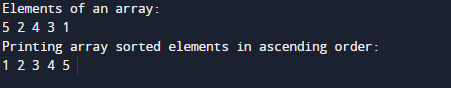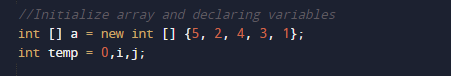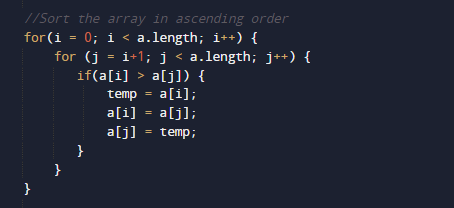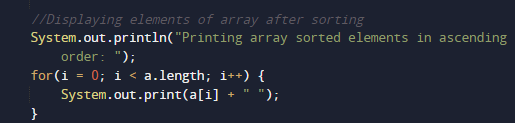# Java Program to Sort Array Elements

In this tutorial you will learn about the Java Program to Sort Array Elements and its application with practical example.

In this tutorial, we will learn to create a Java program that will Sort Array Elements using Java programming.

## Prerequisites

Before starting with this tutorial we assume that you are best aware of the following Java programming topics:

• Java Operators.
• Basic Input and Output
• Class and Object.
• Basic Java programming.
• Array in Java.
• Loops in Java.
• Nested For loops.

## What is array?

An Array is a collection variable (Data)Elements that can store multiple values in a single variable of same type.In Computer Science,an array is a data structure, or simply an array, is a data structure consisting of a collection of elements.

## Sorting.

Sorting is a method in which we sort the given array in Ascending order such that elements will be arranged from smallest to largest.

Entered values->   5 , 1 ,  3 , 4 ,2.
Sorted values ->    1 , 2 , 3 , 4 , 5.
Here  you can see elements will be sorted in  a way that the smallest element will appear in First place here in this case which is  1. The biggest element will appear in Last which in this case is 5.

## Java Program to Sort Array Elements.

In this program we will sort elements of an Array. We would first declared and initialized the required variables. then  we would prompt user to input elements of array .Later we sort  an Array elements in ascending order.

## Outputthe above program, we have first declared and initialized a set variables required in the program.

• a[] = it will hold entered elements.
• i and j= for iteration.
• temp= it hold temporary values.

In our program, we initialize  5 values  in an array , after initialize these values we will sort the elements of an array.First we displayed the original value of an array.And after that we will use selection sort algorithm for sorting the elements of an array by repeatedly finding the minimum element from unsorted part and putting it at the beginning.In every iteration sorted value is arranging in a[i] .At the end the value of sorted array will be printed.In this tutorial, we have learnt to create a Java program that will Sort Array Elements in ascending order.

In this tutorial we have learn about the Java Program to Sort Array Elements and its application with practical example. I hope you will like this tutorial.